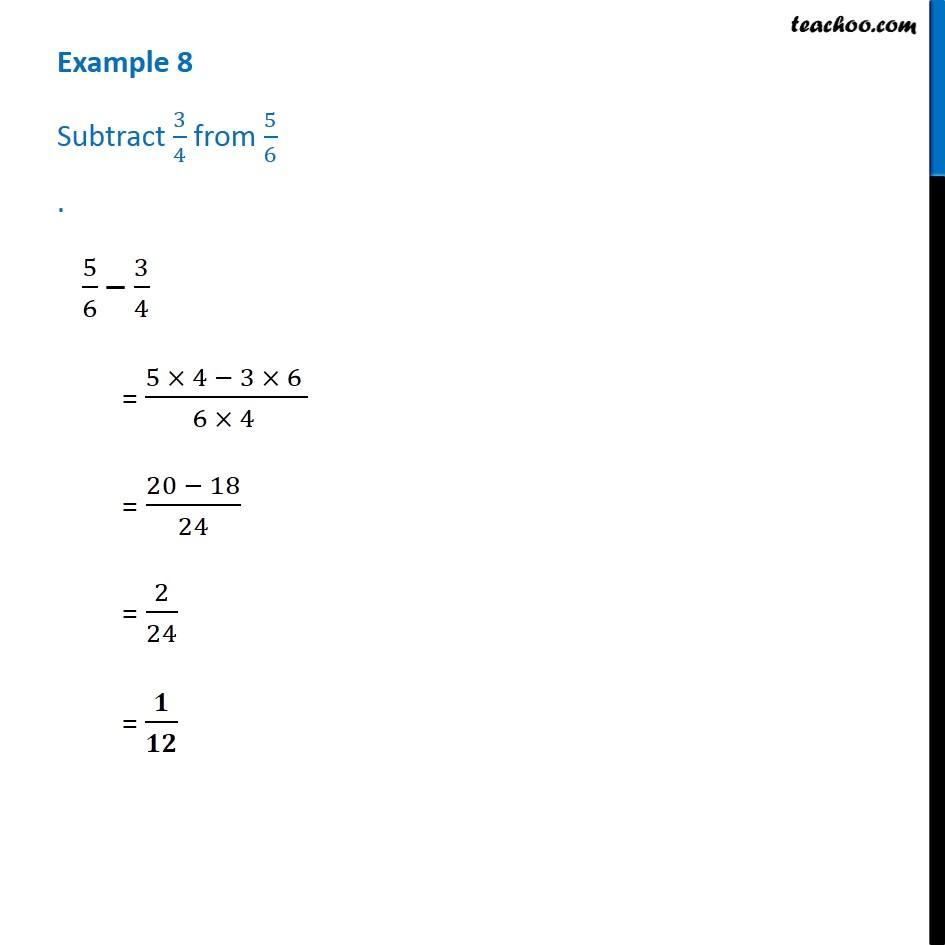1. Chapter 7 Class 6 Fractions
2. Serial order wise
3. Examples

Transcript

Example 8 - Chapter 7 Class 6 Fractions - NCERT Book Subtract 3/4 from 5/6 5/6 – 3/4 = (5 × 4 − 3 × 6) / (6 × 4) = (20 −18)/24 = 2/24 = 1/12

Examples

Chapter 7 Class 6 Fractions
Serial order wise